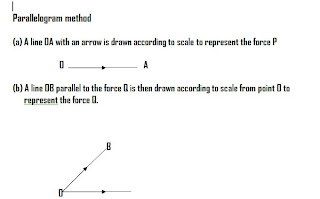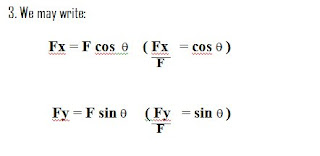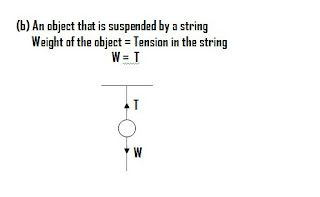## 2009-05-19

### Analysing Forces in Equilibrium

Analysing forces in equilibrium

Vector Addition of Forces

1. A resultant force is a single force that represents the combined effect of two or more
forces in magnitude and direction. The direction of the forces have to be taken into
consideration when forces are added.

2. If the forces act in the same straight line, the resultant is found by simple addition or
subtraction as shown in figure 2.1Resultant force, F = F1 – F2

Figure 2.1

3. The resultant of forces that do not act in the same straight line can be determined by
using the parallelogram law.

4. The parallelogram law states that if two forces acting at a point are represented in size
and direction by the sides of a parallelogram drawn from the point, their resultant is
represented in size and direction by the diagonal of the parallelogram drawn from the
point.Forces in Equilibrium

1. An object is said to be in equilibrium if the object is at rest or is moving with a constant velocity in a straight line.

2. The resultant force that acts on an object is zero if it is in equilibrium. In other words, the forces that act on the object are balanced in all directions.

3. If object is in equilibrium, the resultant force that acts is zero.

4. For two forces acting in the same direction or opposite direction, if the force is not zero, then the object is not in equilibrium.#### 2 comments:Anonymous said...

This is grand!Anonymous said...

Why can' my Physics teacher teach like this!!